How Dice Combinations Work in Craps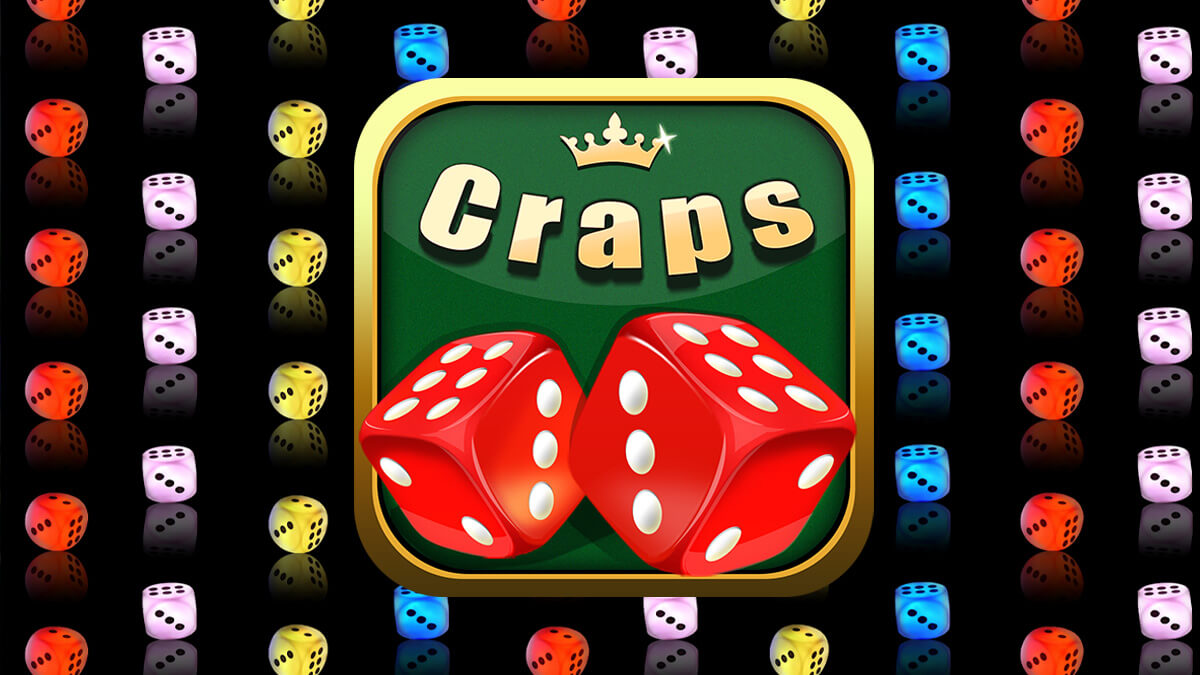Craps is some of the most fun you’ll have in the casino. However, if you want to be an educated craps player, you’ll need to understand some of the probability and odds involved with rolling a pair of dice.

Craps is always played with two dice, each of which is shaped like a cube less than an inch wide. Unlike the dice you’ll buy at your local game store for board games or RPGs, the dice used in craps have sharp edges and pointed corners.

Craps dice are also bigger than the dice you’ll find in a game like Yahtzee or Monopoly. Most of the time, a casino will imprint their logo on the dice they’re using, too. The dice are red, but they’re also translucent, so you can see that there are no weights attached.

Craps shooters often get on hot streaks. If a shooter gets on too hot a streak, the boxman will pause the game to examine the dice to make sure there’s no funny business going on.

The number of possible combinations on a pair of dice is what the game is built around. That’s also the subject of this post: how the dice combinations work in a game of craps.

What Combinations Are There?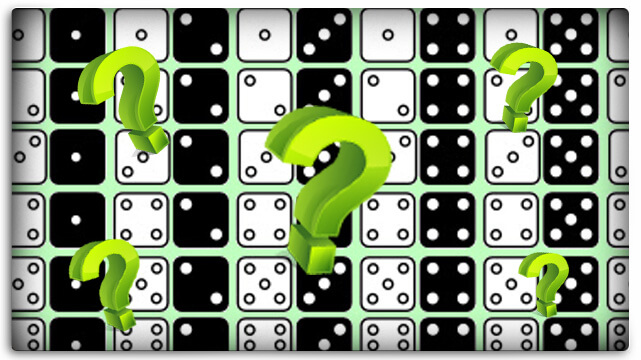Each die has six sides, and they’re numbered from 1 through 6, using dots. If you add the numbers on opposite sides of the dice together, you always get seven. So the 1 and the 6 are opposite each other, the 2 and the 5 are opposite each other, and the 3 and the 4 are opposite each other.

You have a total of 36 possible combinations – you have six possible combinations on one die and six possible combinations on the other die. Out of these 36 possible combinations, you have 11 possible totals.

Here are the possibilities:

1. A total of 2, which can be made up of only one combination: a 1 on each die
2. A total of 3, which can be made up of two different combinations: a 1 on the first die and a 2 on the second die; or a 2 on the first die and a 1 on the second die
3. A total of 4, which can be made up of three different combinations: 1 – 3, 2 – 2, 3 – 1
4. A total of 5, which can be made up of four different combinations: 1 – 4, 2 – 3, 3 – 2, 4 – 1
5. A total of 6, which can be made up of five different combinations: 1 – 5, 2 – 4, 3 – 3, 4 – 2, 5 – 1
6. A total of 7, which can be made up of six different combinations: 1 – 6, 2 – 5, 3 – 4, 4 – 3, 5 – 2, 1 – 6
7. A total of 8, which can be made up of five different combinations: 2 – 6, 3 – 5, 4 – 4, 5 – 3, 6 – 2
8. A total of 9, which can be made up of four different combinations: 3 – 6, 4 – 5, 5 – 4, 6 – 3
9. A total of 10, which can be made up of three different combinations: 4 – 6, 5 – 5, 6 – 4
10. A total of 11, which can be made up of two different combinations: 5 – 6, 6 – 5
11. A total of 12, which be made up of only one combination: a 6 on each die

If you look at this closely, you’ll notice that it makes a symmetrical bell curve. Also, the number of combinations that create a specific total can be divided by 36 to get the probability of getting that total.

A Note on Probability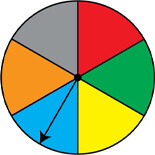Probability is a way to measure how likely it is that an event will occur. For our purposes, an event is a total on two dice.

Probability is just a ratio comparing the number of ways something can happen with the total number of possible events.

For Example:
If you want to know the probability of rolling a 7, you just divide the number of ways you can get a 7 (there are six ways) by the total number of possibilities (36).

Six divided by 36 is the same as 1/6, which is also the same at 16.67%.

When it comes to craps, it’s often useful to use odds as your preferred format for expressing probability. To do that, you just compare the number of ways something can’t happen with the number of ways it can. For example, the odds of rolling a 7 are 5 to 1. They’re actually 30 to six, but you reduce, just like you would a fraction.

You can compare the probability of winning a bet with the payout odds to see what kind of mathematical edge your land-based casino has on a specific bet.

This is the beginning of craps wisdom.

The Point Numbers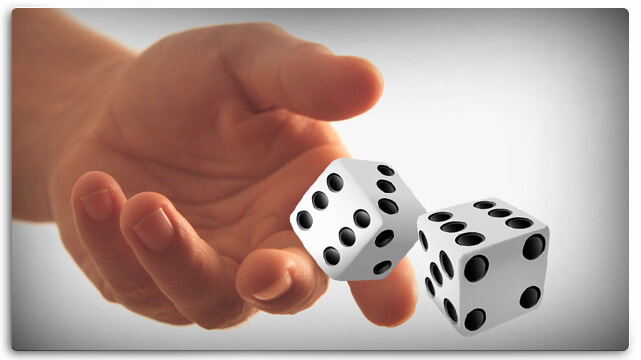The point numbers are 4, 5, 6, 8, 9, and 10.

i
The 4 and the 10 have three possible combinations, making them the least likely of the point numbers to get rolled.

The 5 and the 9 have four possible combinations, and the 6 and the 8 have five possible combinations.

The odds of a 7 coming up before a 4 is easy to calculate. You have six possible combinations totaling 7 versus three possible combinations totaling 4.

That’s 2 to 1 odds.

The odds are the same for rolling a 10.

The odds of a 7 coming up before a 5 (or a 9) are six versus four, or 3 to 2 odds.

The odds of a 7 coming up before a 6 (or an 8) are six versus five, or 6 to 5 odds.

When the shooter makes a point, it’s his job to roll that point total again before rolling a 7.

Now, you know the odds that he’ll succeed.

Proposition Bets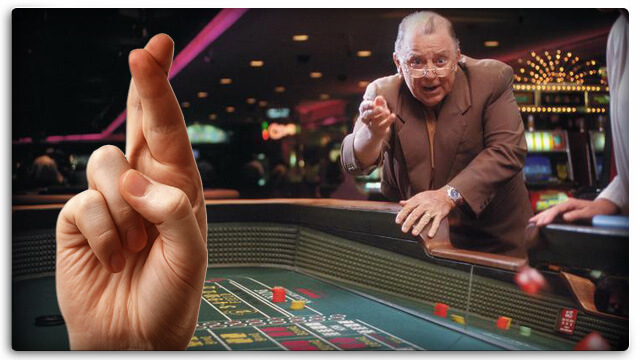One of the worst bets you can make at a craps table is a proposition bet. This is usually a bet on a specific total on the next roll. Depending on the number, the odds might look like the following examples.

If you’re looking at a 2 (snake eyes), the odds of winning are 35 to 1.

If you’re looking at a 12, you face the same odds.

If you’re looking at a 3, the odds of winning are 17 to 1. The same holds true for a total of 11.

If you’re betting on “any 7,” the odds of winning are 5 to 1.

If these bets paid off at those odds, you’d be facing a house edge of zero. If you played long enough, you’d break even or come close to tie.

But the casino isn’t in the business of breaking even. It’s in the money of making a profit.

That’s why they set the payouts for these proposition bets much lower than the odds of winning them.

For Example:
If you bet on the shooter rolling a 2, you face 35 to 1 odds. If you win, though, you only get a 30 to 1 payout.

Statistically, 36 bets of \$100 each would mean losing \$3500 on your 35 losing rolls and winning \$3000 on your one winning roll.

Average that out by 36 rolls of the dice, and you’ve lost an average of \$13.89 per bet or 13.89% of your action.

That’s the house edge, and it’s a huge number.

The “any 7” bet is another proposition bet which is always a one-roll bet, by the way. It’s a bet that the total will be 7 on the next roll.

The odds of winning this one are 5 to 1, but the payout is only 4 to 1.

You can calculate the house edge on this bet easily, too.

Assume six perfect rolls betting \$100 each.

You’ll lose five of those bets for a total of \$500 lost.

On the one bet you win, you’ll get a \$400 payout.

Your net loss over six rolls of the dice is \$100.

Divide that by six, and you can see that the house edge on the any 7 bet is 16.67%.

By the way, when you compare these numbers with the more basic bets at the table – like the pass line bet – it’s easy to see which bets are better.

The pass line bet has a house edge of 1.41%.

On average, over enough rolls of the dice, you should lose \$1.41 every time you place a \$100 bet on the pass line.

But if you bet that same \$100 on any 7, you’d lose \$16.67.

Which one of those sounds like the better bet to you?

Conclusion

That’s as good an introduction to the dice combinations in craps as you’ll find. Once you understand the math in this blog post, you can figure out everything you need to know about every bet at the table.